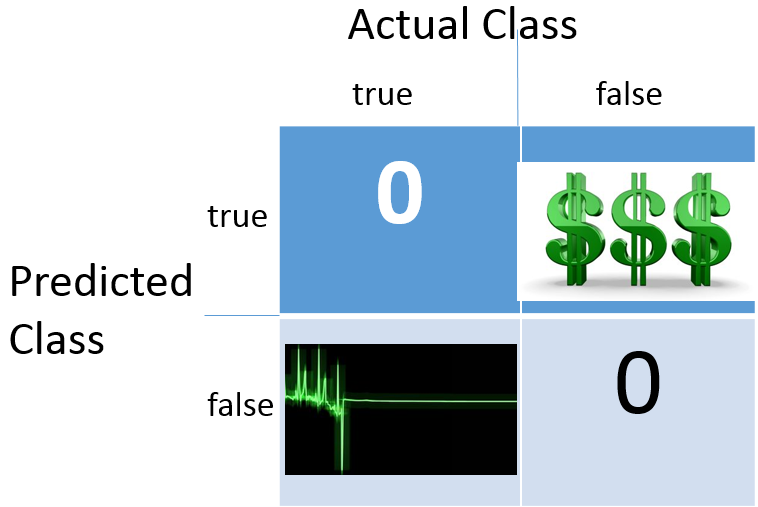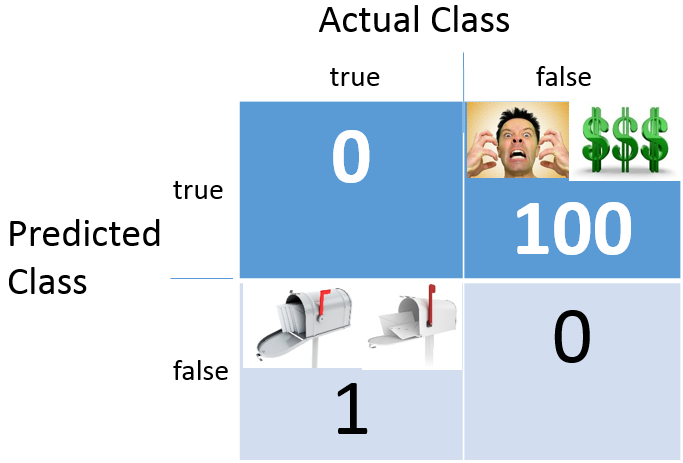# Cost-sensitive Statistical Relational Learning

In this project, we consider the problem of incorporating the domain knowledge on different weights of positive samples and negative samples. One of the motivations is the class-imbalance situation in many relational domains where the classifier boundary could be easily dominated by the majority class and overfitting on its outliers. Hence, it is essential to steer the training process toward focusing more on the minority class by assigning different costs on false positive and false negative samples. Besides the requirement enforced by such data properties, there are also practical demands in certain domains, such as the diagnosis problem in medical domains, the quality checking in manufacturing data, the recommendation prediction in recommender systems, etc.

The common approach for dealing with this problem is sampling approach, either sub-sampling of the majority class or over-sampling on the minority class. We proposed a soft margin learning approach on the basis of relational functional gradient boosting. Our approach allows explicit tunning the trade-off between false positive rate and false negative rate during the learning process by including two paramters into the objective function, which control the weights of false positives and false negatives respectively.

# What domains are applicable?

## Class-Imbalanced Domains

[Yang et al. 2014]Class-Imbalance is a phenomenal problem in a lot of domains, especially for statistical relational learning problems where the number of the ground substitutions for a logical predicate is exponential in the number of the instances for the logical variables and among them only a few substitutions are true.

## False Negatives Cost More

[MacLeod et al. 2016]Domains where the cost for false negative prediction is much more than that of the false positive prediction. For example, in medical diagnosis, the false positive prediction may just lead to few more clinical tests while the false negative prediction could cost the patient’s life.

## False Positives Cost More

[Yang et al. 2017]Domains where the false positive prediction is more unfavorable. For example, in recommendation systems, one would rather overlook some of the candidate items that could match the users (false negatives) than send out numerous spam emails to the users with inappropriate recommendations (false positives).

# How to use this package?

The package includes the pre-processing code for standard machine learning input data, the Soft-Margin RFGB code and the code for calculating the measurements of evaluating the performance of learning algorithms for class-imbalance problems.

## Data Pre-Processing

For standard machine learning problems, just run the python script ConvertStandardData.py in the package to convert the flat table into the input data format that Soft-Margin RFGB can take. The package also contains a sample data set–heart disease data from UCI Machine Learning Repository. Users can use this script to convert any data set from UCI Machine Learning Repository by simply adding the variable names in the first row.

A sample usage is as below:

``````\$ python ConvertStandardData.py filename=PATH/TO/YOUR/DATA/DATA.csv \
> target=TargetVariable \
> Discretize='feature1':[threshold list],'feature2':['value', Nclass],'feature3' ['quantile',Nclass] \
> TestRatio=0.1 ``````

The optional arguments are Discretize and TestRatio .

Use Discretize if one wants to discretize the continuous-valued variables. There are three options: i. assign categorical values based on the thresholds given as a list; ii. categorize into N classes based on values by specifying ['value', Nclass] ; iii. discretize into N bins based on sample quantiles by specifying ['quantile', Nclass]. If not given, numerical variables will be written out in their original value types.

Use TestRatio to specify how you want to split the data into training and test sets. If not assigned, 80% of the samples will be assigned to the training and 20% to test.

For relational data sets, please refer to Mode Guide for more sophisticated designs of logic predicates.

## Run Soft-Margin RFGB

Here is a simple example on how to use the Soft-Margin RFGB code.

``````\$ java -cp SoftBoosting.jar edu.wisc.cs.Boosting.RDN.RunBoostedRDN \
> -target num \
> -l -train SampleData/OutputDataForSoft-RFGB/HD/train/ \
> -i -test SampleData/OutputDataForSoft-RFGB/HD/test/ \
> -alpha 2 \
> -beta -1 ``````

The parameter alpha controls the cost of false negative samples while beta controls the cost of false positive samples. When the parameter (alpha or beta) is set positive, it assigns more weights on the miss-classified positive or negative samples, whearas when it is negative, it allows the model to put more tolerance on the the miss-classified positive or negative samples. When they are both zero, it is equivalent to the standard RFGB, i.e. false positive and false negative have uniform cost.

## Evaluation Metrics For Class-Imbalanced Data

Standard evaluation metrics for the prediction performance include the use of accuracy, Area Under ROC or PR curves (AUC-ROC or AUC-PR), F1 score, etc., which measure accuracy with balanced weight between positive and negative examples. However, in the cost-sensitive learning, the model should identify as many important cases as possible as long as the accuracy on predicting the less importance class stays within a reasonable range. To better evaluate the performance of different algorithms for learning with class-imbalanced data, we employed F-beta measure and weighted AUC-ROC. For F-beta measure, beta controls the importance of Precision and Recall. When beta > 1, F-beta measure is recall dominated, while as 0< beta < 1 F-beta measure is precision dominated.

More details about the algorithm and parameter settings can be found in [Yang et al. 2014]. Please use the following citation to acknowledge the contribution made by this package to your research. 1

1. Shuo Yang, Tushar Khot, Kristian Kersting, Gautam Kunapuli, Kris Hauser and Sriraam Natarajan, Learning from Imbalanced Data in Relational Domains: A Soft Margin Approach, International Conference on Data Mining (ICDM), 2014. ^##### Shuo Yang
###### Senior Research Scientist

My research interests include machine learning and its application in health and other real-world problems.